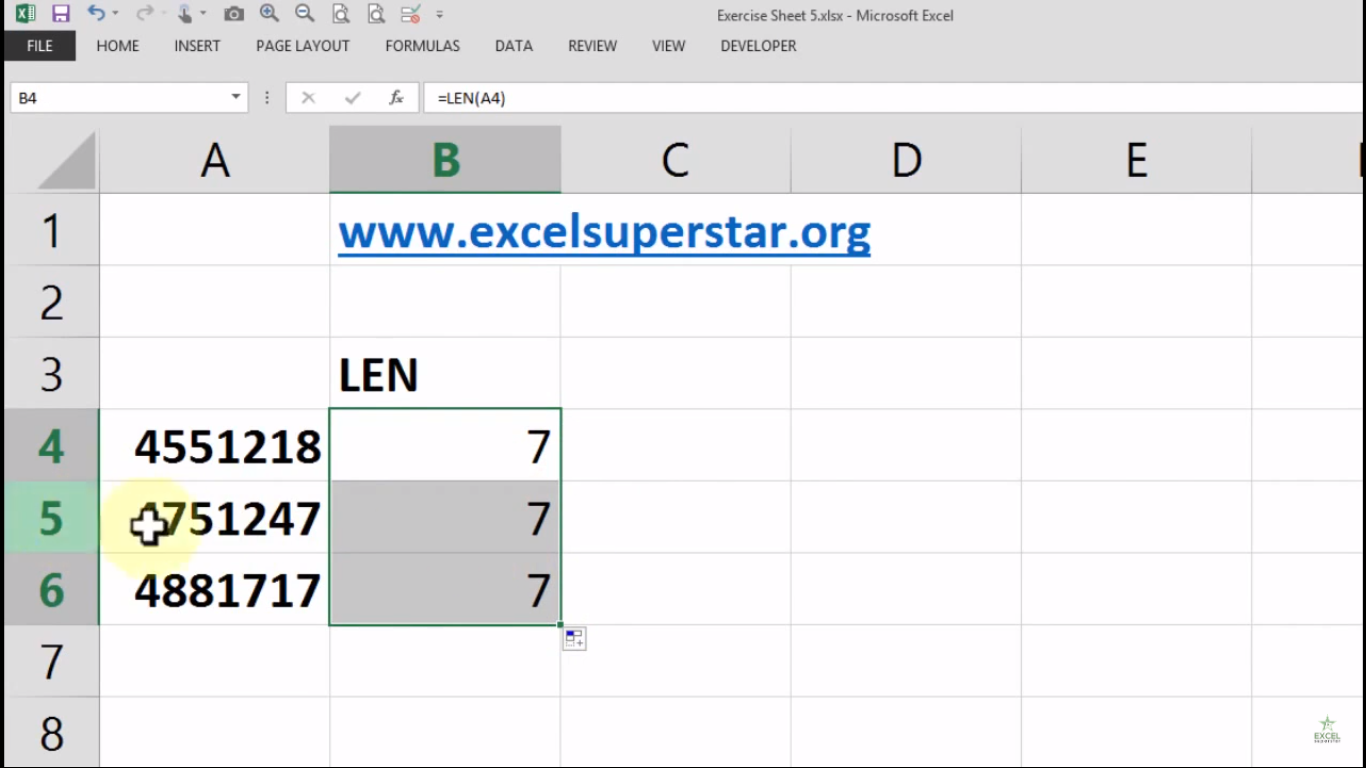# Excel LEN FunctionIn this tutorial we will learn about How to use LEN Function in Excel

Example: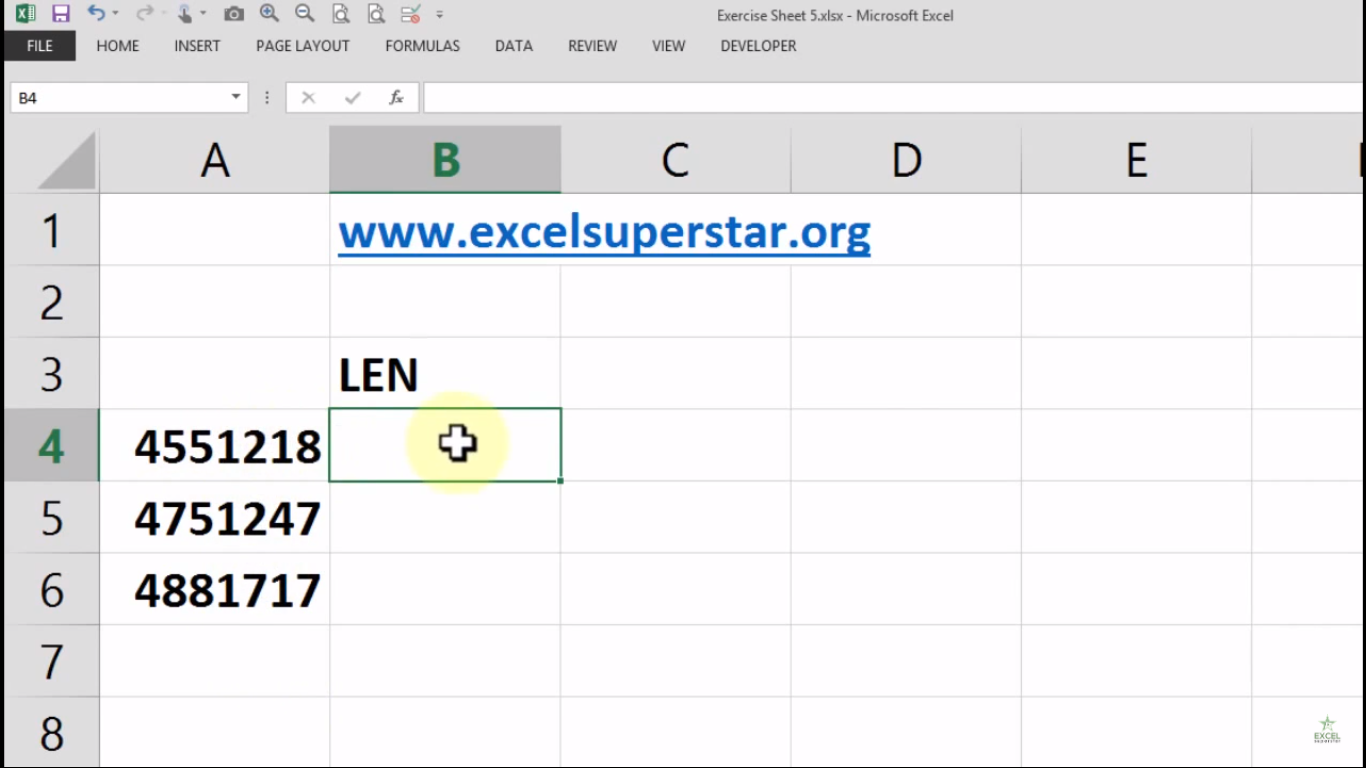I have some digits written inside the cells and want to count the digits inside a particular cell

So here we will be using LEN Function in Excel to count the digits inside a particular cell

1. Write =LEN and press the Tab Key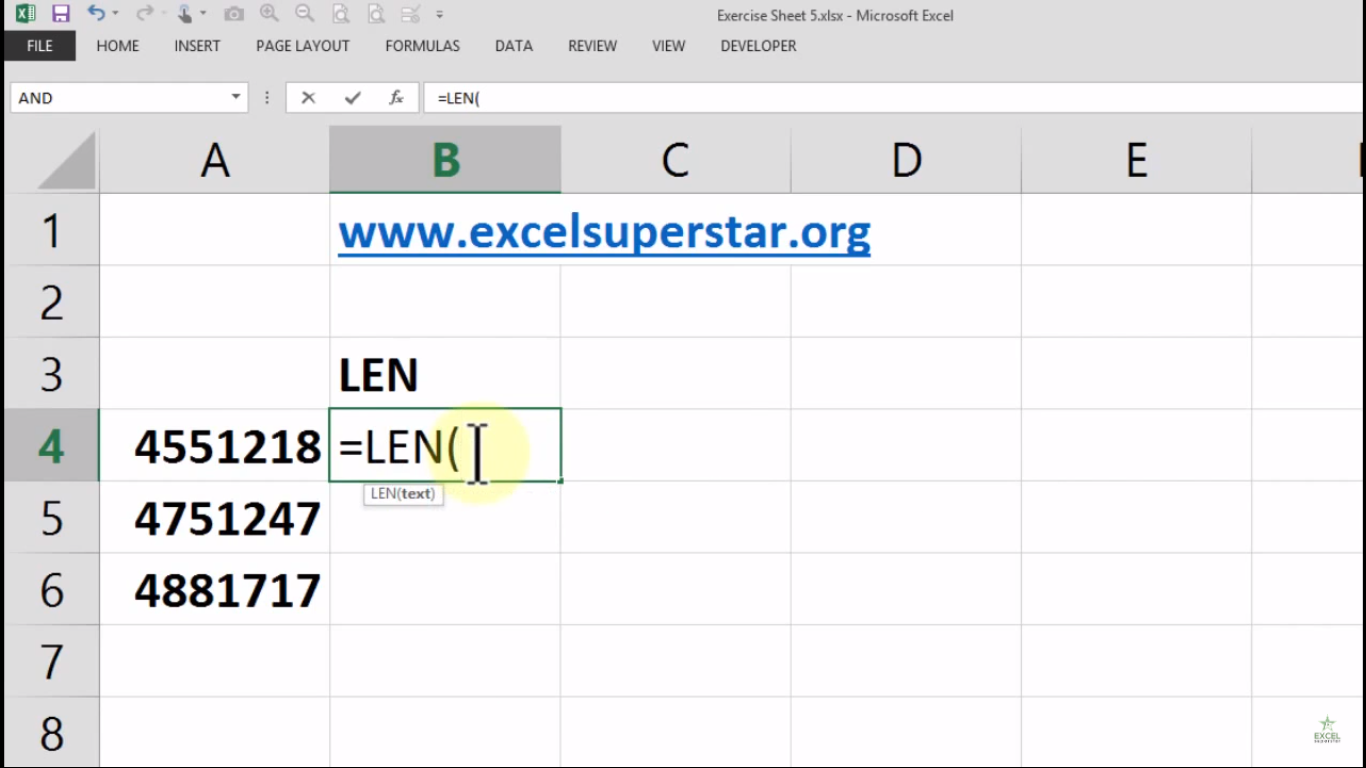A single parameter text will appear which means the text for which to calculate length

2. Select the cell as text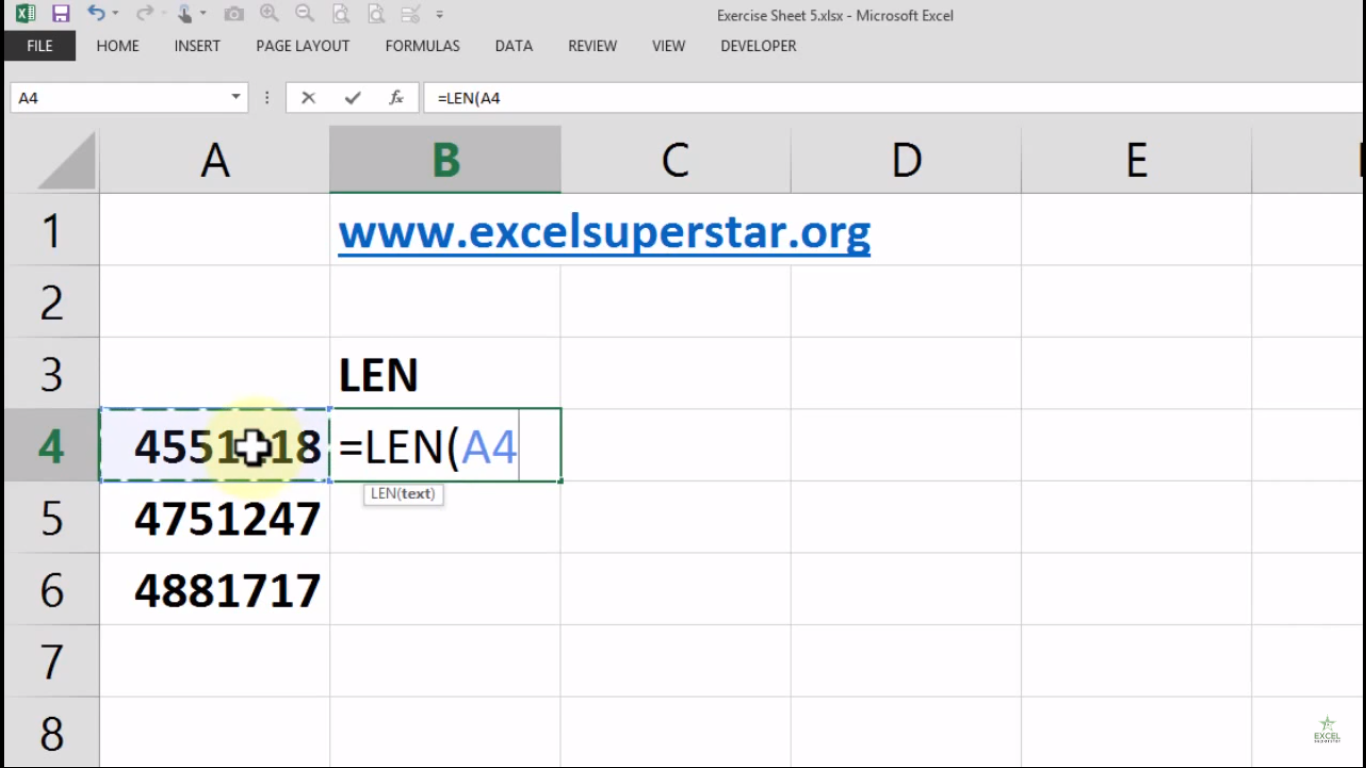3. Close the bracket and press Enter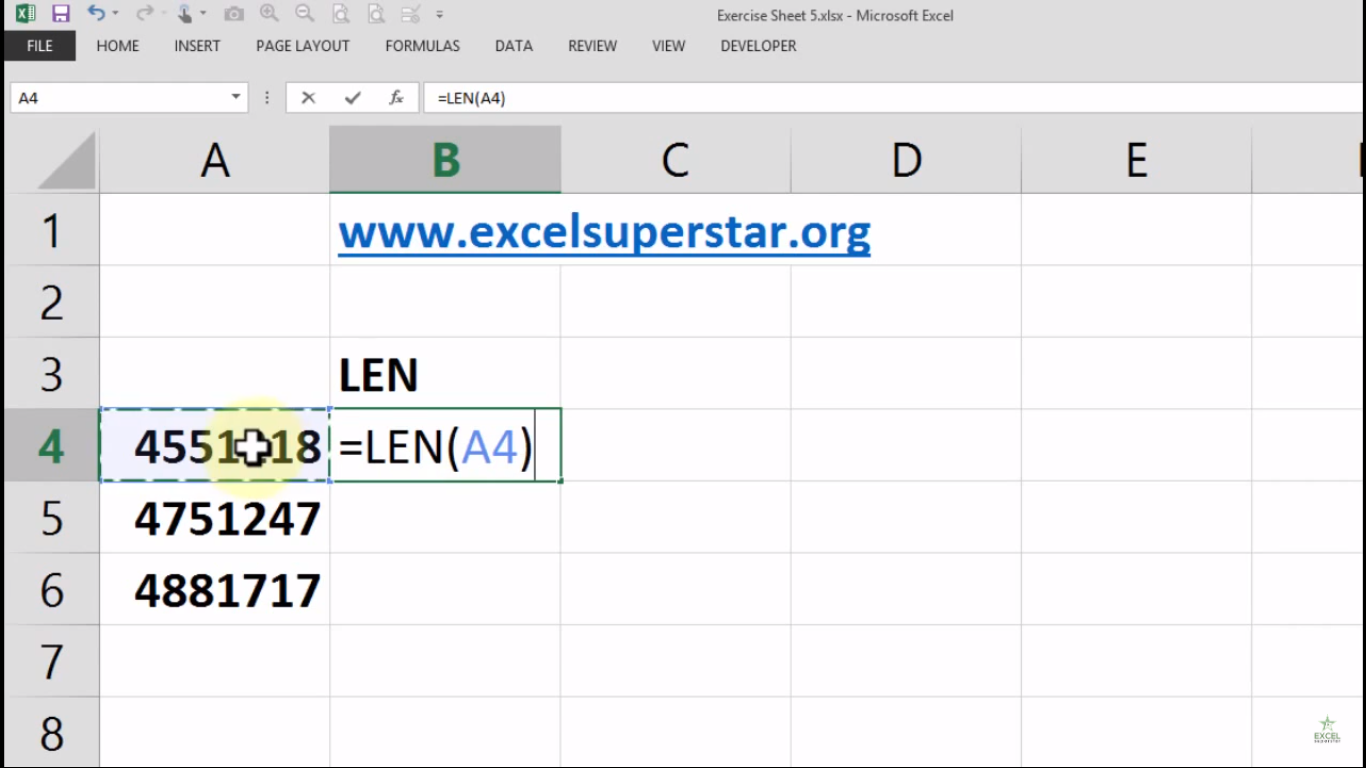4. Excel will count the digits inside a particular cell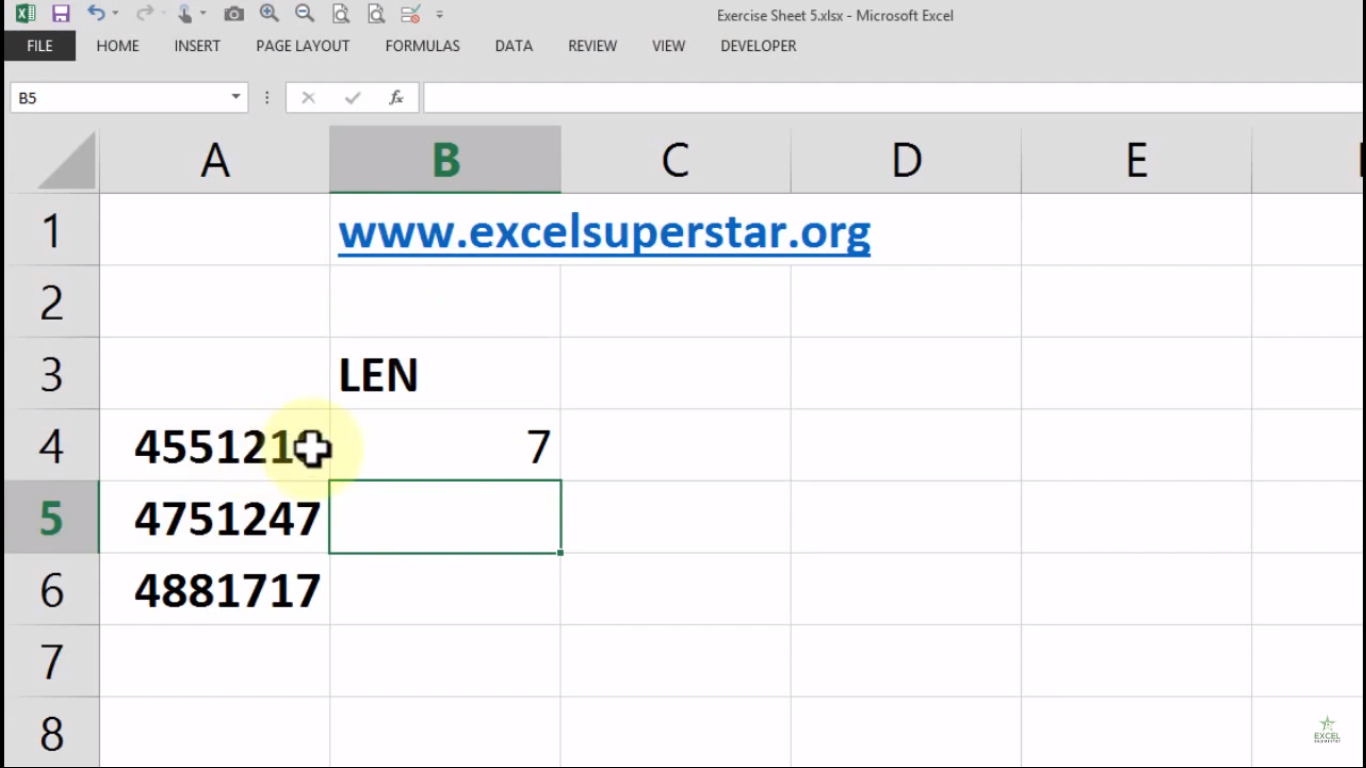Note – If there is a space between the numbers then also it will be considered as character

5. Drag the cell to the bottom to count digits of other cell